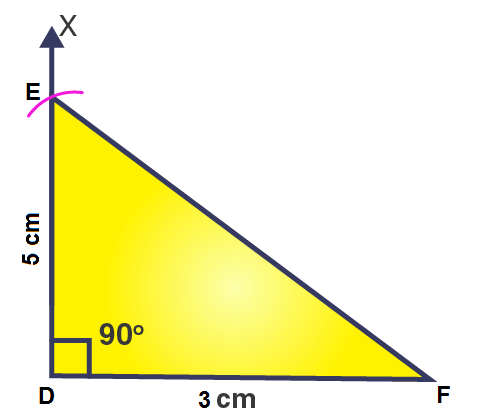# Construct ΔDEF such that DE = 5 cm, DF = 3 cm and m∠EDF = 90o.

Solution>>

The steps for construction are given below:

1. Draw a line segment DF = 3 cm.

2. At point D, draw a ray DX to making an angle of 90o i.e. ∠XDF = 90o.

3. Along DX, set off DE = 5cm.

4. Join EF.

Then, ΔEDF obtained is the required right angled triangle.(0)(0)